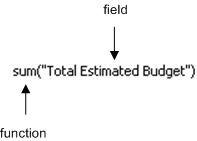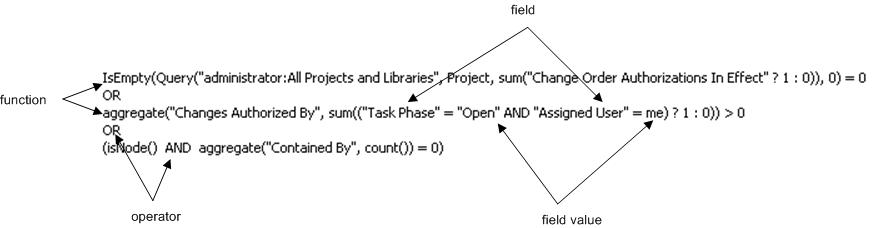> > Computed Expression Rules

Computed Expression Rules
The rules for writing computed expressions are similar to the rules for writing expressions in most programming languages. Computed expressions use a specific syntax and operators, constants, functions, and Windchill RV&S fields to perform calculations.
For example, the following diagram displays a simple computed expression involving one function and one field to perform an aggregate calculation:The computed expression is used in a chart to calculate the sum total of a field that appears in several items.
The following diagram displays a more complex computed expression that strings together several functions, fields, field values, and operators to perform a calculation in a computed field:For illustration purposes, the diagram outlines some of the functions, fields, field values, and operators.The computed expression in the computed field determines if the item is a valid Change Order.Before you create a computed expression, review the following key considerations to understand acceptable syntax, operators, and constants.
Key Considerations
General
It is outside the scope of this guide to provide explicit instructions on how to write a computed expression. However, this guide does provide several examples of simple and complex computed expressions to illustrate some of the ways you can perform calculations in fields, charts, and reports.
If you do not provide proper syntax for a computed expression, Windchill RV&S displays a detailed error message when you save the computed expression.
Syntax
To specify field names, surround the field name with double quotation marks, for example, "Actual Cost".
Spaces and special characters are ignored, except within quotations, for example, "Defect Count".
Identifiers followed by “(“ (a parenthesis) are considered functions. Identifiers not followed by a parenthesis are considered field names.
Computed fields are valid as fields used in another computed field or computed expression; however, they may not recurse.
Arithmetic Operators
Computed expressions support the following arithmetic operators:
* (multiplication)
/ (division)
- (subtraction)
- (unary negation)
Boolean-exp?true_exp:false_exp (conditional ternary)
Boolean Operators
Computed expressions support the following boolean operators:
== (equal)
= (contains)
!= (not equal)
<> (does not contain)
< (less than)
> (greater than)
<= (less than or equal to)
>=(greater than or equal to)
and
or
expression boolean-operator expression
For boolean fields, two keywords are boolean constants: true and false. When a computed field is marked against a boolean field, the result of the computed expression must be a boolean value, for example:
Empty Fields
In Windchill RV&S, a field without a value is referred to as empty. A computed expression that includes an empty field or an ambiguous field will return an empty value, except in aggregate expressions.
For example, to determine if the Project End Date date field is empty, type:
"Project End Date"-today() > 0
or
"Project End Date" - today() <=0? false:true
To specify an empty field value as zero, use the isEmpty function. For example:
isEmpty("QA Time", 0) + isEmpty("Development Time", 0)
allows you to add two time durations and retrieve a result, even if one of the values is empty.If a computed field can contain an ambiguous computation, as a best practice, PTC recommends wrapping the expression in the IsEmpty() function. This ensures that any empty computed field values stored to history are as a result of an ambiguous computed expression.
Dates, Times, and Timezones
Dates and dates/times are considered separate date types in computed expressions, and cannot be assigned to one another.
Displayed date fields do not change based on the time zone that a user is in; however, displayed date/time fields and time entries vary based on the time zone that a user is in.
Computed expressions return dates/times in the Windchill RV&S client’s time zone and perform calculations in the Windchill RV&S server’s time zone, where appropriate.
Computed expressions do not include a function for converting date/time information to date-only. This applies in all cases where an expression returns a timestamp (date plus time) value. Where date/time information is required, use the computed expression with a timestamp field.
Date expressions accept all standardized date and time stamp formats.
The following operations are supported with time stamps in computed expressions:You cannot mix dates and timestamps.
timestamp + integer constant; integer constant + timestamp
timestamp - integer constant
timestamp("timestamp-constant")
timestamp - timestamp
timestamps can be subtracted or added to a constant integer, for example, now()+5 or now() - 5
Integer constants are treated in units of days; for example, timestamp + integer constant takes the specified time and adds a specified number of days. When subtracting two timestamps, the number of days between the two dates rounded to the nearest day is the result. Using other operators or a floating point results in an error.
User and Group Fields
You can compare single- and multi-valued user and group fields in computed expressions, for example, "Assigned User" = jriley, or "Assigned Group" = Development. By creating a computed expression that includes a user or group field comparison, you can incorporate the result of the computed field into an editability rule.
You can also compare two user fields in a computed expression, for example, “Assigned User” = “Created By”. A logical value or true or false is returned.
You can also use the symbolic me user name to indicate the user retrieving the item.